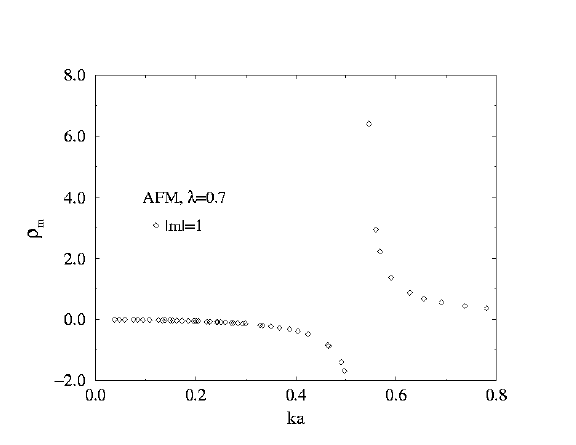## Magnetic Vortices and Spinwaves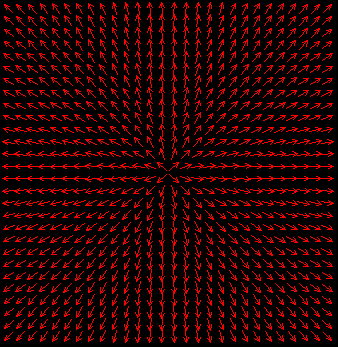These are data showing the spin-wave-functions of the spinwaves in the presence of an in-plane magnetic vortex in a two-dimensional magnet. The spinwave modes were found by a relaxation method, which gives both the wavefunctions and frequencies of some of the lowest modes. We considered ferromagnetic and antiferromagnetic models. A vortex in a ferromagnet is shown to the right.

### Easy-Plane Magnetic Model

The nearest-neighbor Hamiltonian has easy-plane anisotropy parameter lambda, in a form

Hn,m = J * Sn . Sm

where the Sn are three-component spins and J is a diagonal matrix:

```
1   0   0

J  =   0   1   0

0   0  lambda

```
We consider a square lattice of spins, with a circular boundary at radius R, and 0 <= lambda < lambda_c, where lambda_c = 0.7034 is the critical anisotropy parameter. For this anisotropy range, the vortex configuration is purely in the xy-plane (in-plane vortex). Fixed boundary conditions were used.

### Spinwave Modes

The modes are labeled by a principle quantum number 'n' and azimuthal quantum number 'm', and represent the possible forms of oscillation of the spin field, on top of the original vortex structure. omega is the oscillation frequency. The quantum numbers are the numbers of nodal lines in the radial and azimuthal directions, respectively.

On the mode diagrams below, blue arrows represent the magnitude (arrow length) and phase (arrow direction) of the oscillations of in-plane (xy) spin components. Red arrows represent the magnitude (arrow length) and phase (arrow direction) of the out-of-plane (z) spin components.

### Scattering and S-matrix

The S-matrix is derived from fitting the wavefunctions to a sum of incoming plus outgoing waves, with amplitude of the outgoing waves relative to the incoming ones being called 'S'. The in-plane part of the wavefunctions was fit to a form like

Psi = A [ Jm(kr) + rhom(k) Ym(kr) ]

where Jm and Ym are Bessel functions, and A and rhom(k) are fitting constants. 'k' is the wavevector for a spinwave, which can be derived from the spinwave dispersion relation because we know the spinwave frequency. Sm(k) is then derived from rhom(k), by a simple formula,

Sm(k) = [1 - i rhom(k) ]/[1 + i rhom(k) ]

Below we show some plots of the results obtained for rhom(k), for only the acoustic branch spinwaves (not for the local mode of the AFM model, which is from the optical branch).

### Ferromagnet at lambda=0.7

This is anisotropy parameter just below the critical value of lambda_c=0.7034, above which the static vortex would possess an out-of-easy-plane component. Here, the lowest mode, n=0, m=0, has frequency nearly independent of system radius. This mode is the vortex instability mode, and is quite local in out-of-plane oscillations, but extended in in-plane oscillations. Note also the interesting singularities in the scattering for m=0, but smoother behavior at m=1, 2.

#### Vortex-Spinwave Scattering Modes for Ferromagnet R=28, lambda=0.7

n=2, m=0,
n=1, m=1,
n=0, m=3,
n=1, m=0,
n=0, m=2,
n=0, m=2,
(m=2 states not exactly degenerate, due to lattice)
n=0, m=1,
(translational mode)
n=0, m=0,
(quasi-local mode or vortex-instability mode)

#### Spinwave frequencies vs. system radius R, Ferromagnet, lambda=0.7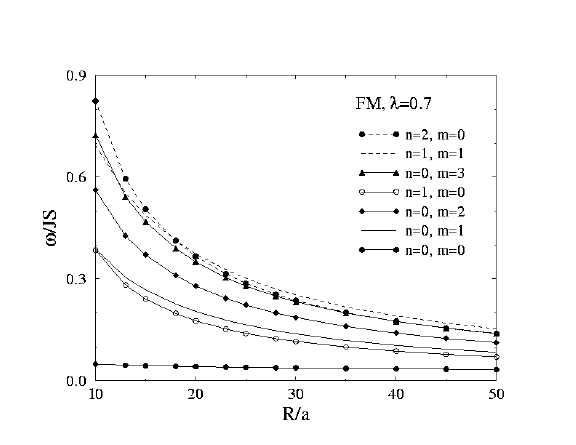#### Scattering Rates for Ferromagnet lambda=0.7 m=0, 1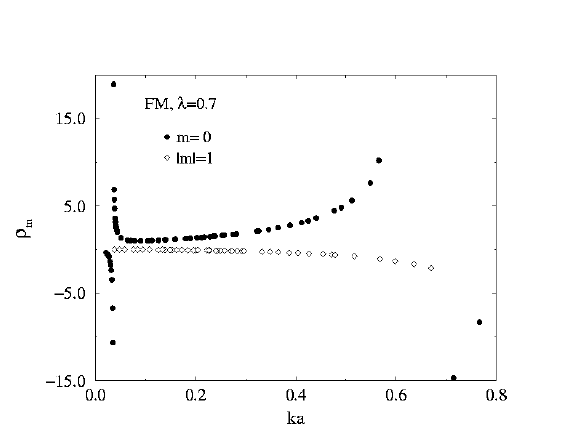#### Scattering Rates for Ferromagnet lambda=0.7 m=2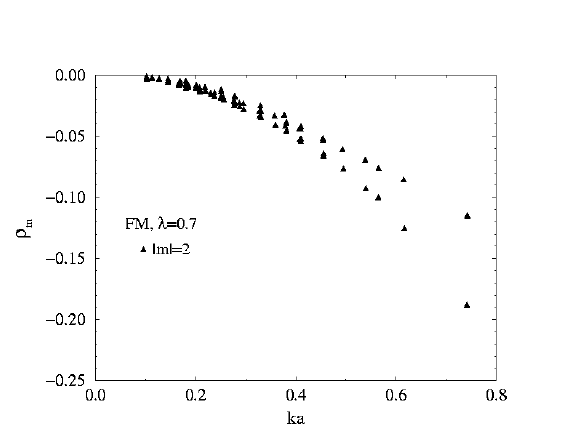### Ferromagnet at lambda=0.5

Farther from the critical anisotropy. The n=0, m=0 mode now has considerable frequency change with system radius. Scattering at m=0 is smoother, no singularities appeared over the range of k studied.

#### Vortex-Spinwave Scattering Modes for Ferromagnet R=28, lambda=0.5

n=2, m=0,
n=1, m=1,
n=0, m=3,
n=1, m=0,
n=0, m=2,
n=0, m=2,
(m=2 states not exactly degenerate, due to lattice)
n=0, m=1,
(translational mode)
n=0, m=0,
(quasi-local mode or vortex-instability mode)

#### Spinwave frequencies vs. system radius R, Ferromagnet, lambda=0.5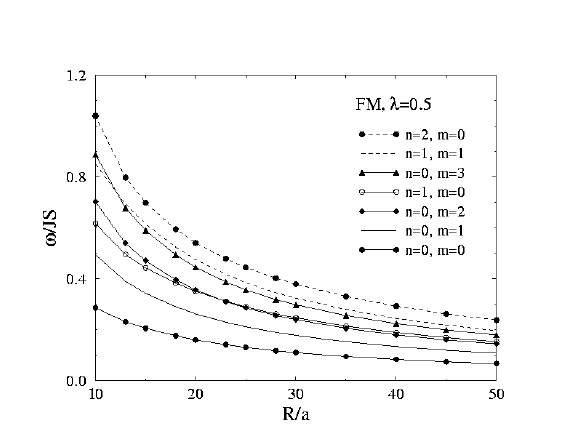#### Scattering Rates for Ferromagnet lambda=0.5 m=0, 1, 2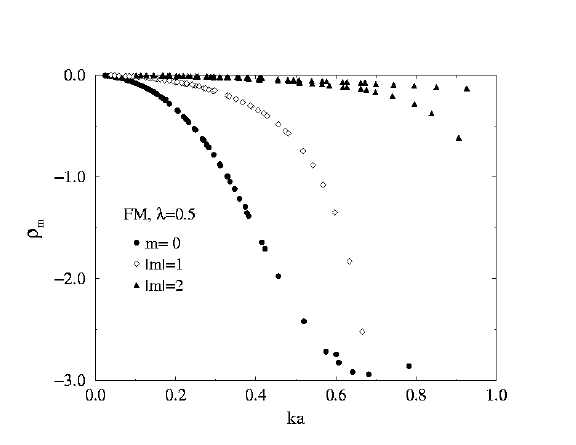### FM or AFM at lambda=0

At this anisotropy value, the eigenvalue problem for the spinwaves is the same for FM and AFM models, so they have identical spinwave frequencies and wavefunctions. As a result, even the scattering rates rhom(k) are the same.

Also note: the n=0, m=0 mode here evolves into the vortex instability mode as lambda approaches lambda_c for the FM model. However, this mode does nothing interesting as lambda approaches lambda_c for the AFM model. The truly local mode of the AFM model actually comes down from a higher frequency mode taken out of the optical spinwave branch. See the results for lambda=0.7 for AFM model, below.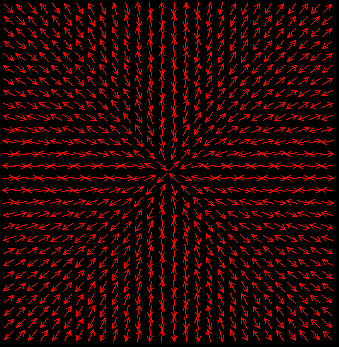An in-plane vortex in an antiferromagnet

#### Vortex-Spinwave Scattering Modes for Ferromagnet or Antiferromagnet R=28, lambda=0.0

(These have identical spectra.)

n=3, m=0,
n=2, m=1,
n=0, m=5,
n=2, m=0,
n=1, m=1,
n=0, m=3,
n=1, m=0,
n=0, m=2,
n=0, m=2,
(m=2 states not exactly degenerate, due to lattice)
n=0, m=1,
(translational mode)
n=0, m=0,
(lowest mode --> FM quasi-local mode)

#### Spinwave frequencies vs. system radius R, Ferromanget or Antiferromagnet, lambda=0.0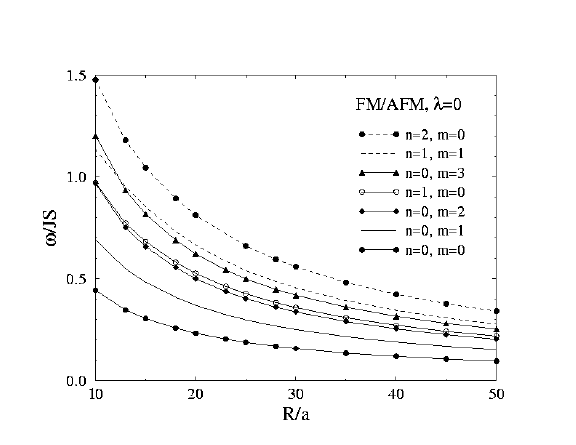#### Scattering Rates for Ferromagnet or Antiferromagnet lambda=0.0 m=0, 1, 2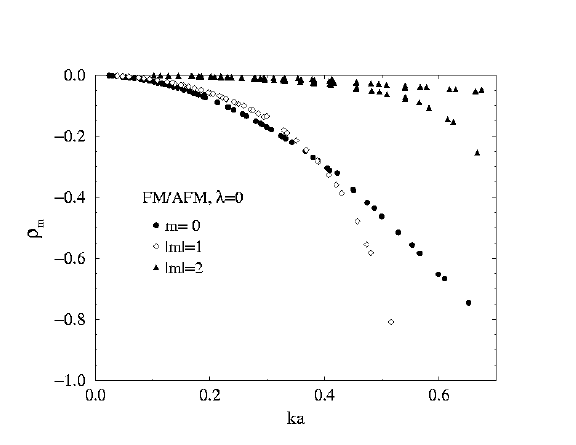### Antiferromagnet at lambda=0.5

Check out the local mode that appears near omega/JS = 1, independently of the system radius (purple curve on figure). The other modes tend to have frequencies diminishing as 1/R. The red arrows displayed in the wavefunction for this mode show that oscillations of the out-of-easy-plane components are becoming important. The mode here is actually a mixture of m=0 and m=2 components.

#### Vortex-Spinwave Scattering Modes for AntiFerromagnet R=28, lambda=0.5

n=0, m=0,
(truly local mode or vortex-instability mode, optical branch)
n=2, m=0,
n=1, m=1,
n=0, m=3,
n=1, m=0,
n=0, m=2,
n=0, m=2,
(m=2 states not exactly degenerate, due to lattice)
n=0, m=1,
(translational mode)
n=0, m=0,
(lowest mode, extended, acoustic branch)

#### Spinwave frequencies vs. system radius R, Antiferromagnet, lambda=0.5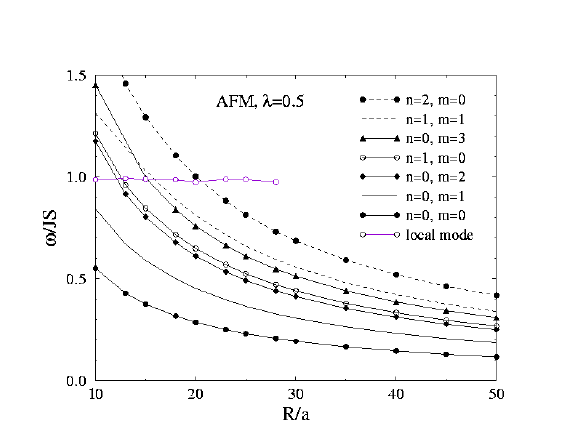#### Scattering Rates for AntiFerromagnet lambda=0.5 m=0, 2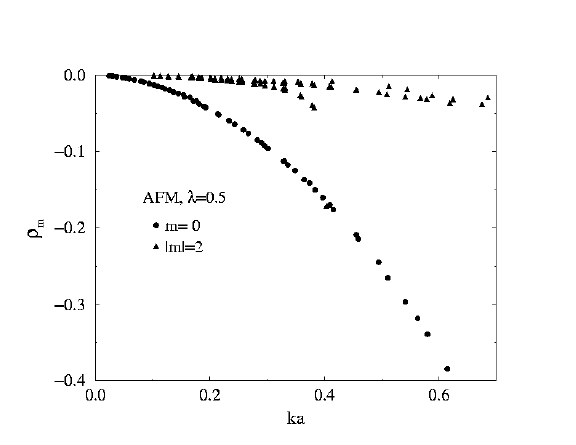#### Scattering Rates for AntiFerromagnet lambda=0.5 m=1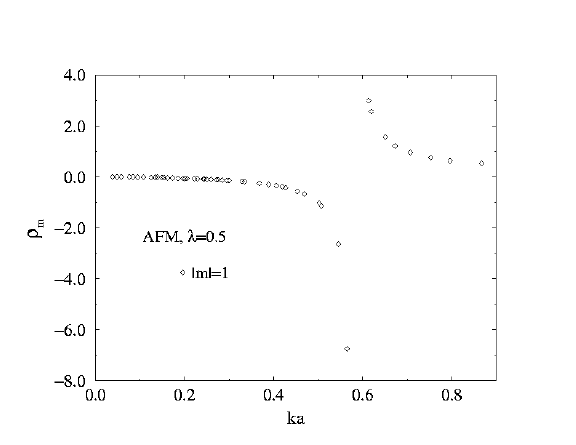### Antiferromagnet at lambda=0.7

Now the local mode has moved down near near omega/JS = 0.143, independently of the system radius (purple curve on figure). The other modes still tend to have frequencies diminishing as 1/R. The stronger red arrows displayed in the wavefunction for this mode (compared to how it looked at lambda=0.5) show that oscillations of the out-of-easy-plane components dominate in this mode. Thus it is also associated with how this in-plane vortex tries to become an out-of-plane vortex as lambda approaches lambda_c=0.7034 .

Both here and at lambda=0.5 a singularity appears in the m=1 scattering at fairly large wavevector.

#### Vortex-Spinwave Scattering Modes for AntiFerromagnet R=28, lambda=0.7

n=2, m=0,
n=1, m=1,
n=0, m=3,
n=1, m=0,
n=0, m=2,
n=0, m=2,
(m=2 states not exactly degenerate, due to lattice)
n=0, m=1,
(translational mode)
n=0, m=0,
(extended mode, acoustic branch)
n=0, m=0,
(truly local mode or vortex-instability mode, optical branch)

#### Spinwave frequencies vs. system radius R, Antiferromagnet, lambda=0.7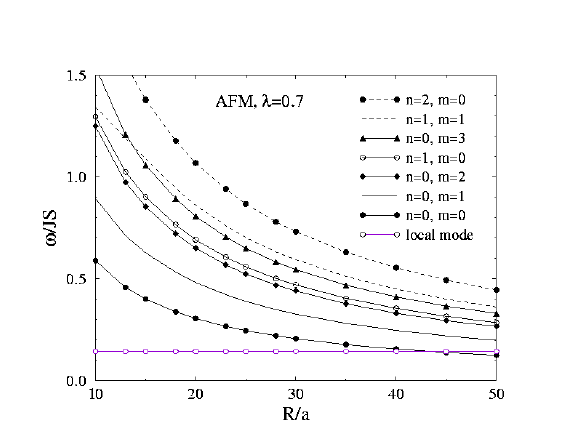#### Scattering Rates for AntiFerromagnet lambda=0.7 m=0, 2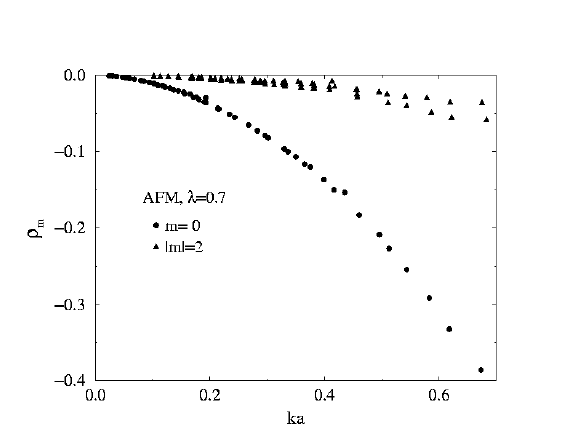#### Scattering Rates for AntiFerromagnet lambda=0.7 m=1